# Antiparallelogram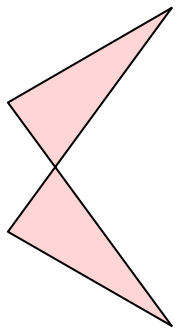An antiparallelogram

In geometry, an antiparallelogram is a quadrilateral having, like a parallelogram, two opposite pairs of equal-length sides, but in which the sides of one pair cross each other. The longer of the two pairs will always be the one that crosses. Antiparallelograms are also called contraparallelograms or crossed parallelograms.

An antiparallelogram is a special case of a crossed quadrilateral, which has generally unequal edges. A special form of the antiparallelogram is a crossed rectangle, in which two opposite edges are parallel.

## Properties

Every antiparallelogram has an axis of symmetry through its crossing point. Because of this symmetry, it has two pairs of equal angles as well as two pairs of equal sides. Together with the kites and the isosceles trapezoids, antiparallelograms form one of three basic classes of quadrilaterals with a symmetry axis. The convex hull of an antiparallelogram is an isosceles trapezoid, and every antiparallelogram may be formed from the non-parallel sides (or either pair of parallel sides in case of a rectangle) and diagonals of an isosceles trapezoid.

Every antiparallelogram is a cyclic quadrilateral, meaning that its four vertices all lie on a single circle.

## Uniform polyhedra and their duals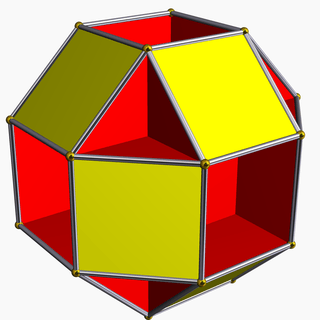The small rhombihexahedron. Slicing off any vertex of this shape gives an antiparallelogram cross-section as the vertex figure.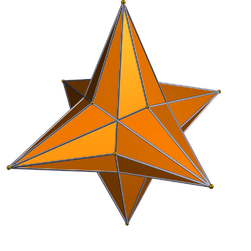The small rhombihexacron, a polyhedron with antiparallelograms (formed by pairs of coplanar triangles) as its faces

Several nonconvex uniform polyhedra, including the tetrahemihexahedron, cubohemioctahedron, octahemioctahedron, small rhombihexahedron, small icosihemidodecahedron, and small dodecahemidodecahedron, have antiparallelograms as their vertex figures, the cross-sections formed by slicing the polyhedron by a plane that passes near a vertex, perpendicularly to the axis between the vertex and the center.

For uniform polyhedra of this type in which the faces do not pass through the center point of the polyhedron, the dual polyhedron has antiparallelograms as its faces; examples of dual uniform polyhedra with antiparallelogram faces include the small rhombihexacron, the great rhombihexacron, the small rhombidodecacron, the great rhombidodecacron, the small dodecicosacron, and the great dodecicosacron. The antiparallelograms that form the faces of these dual uniform polyhedra are the same antiparallelograms that form the vertex figure of the original uniform polyhedron.

The antiparallelogram has been used as a form of four-bar linkage, in which four rigid beams of fixed length (the four sides of the antiparallelogram) may rotate with respect to each other at joints placed at the four vertices of the antiparallelogram. In this context it is also called a butterfly or bow-tie linkage. As a linkage, it has a point of instability in which it can be converted into a parallelogram and vice versa.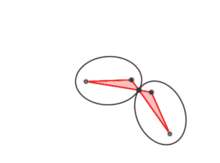Fixing the short edge of an antiparallelogram linkage causes the crossing point to trace out an ellipse.

If one of the short (uncrossed) edges of an antiparallelogram linkage is fixed in place, and the remaining linkage moves freely, then the crossing point of the antiparallelogram traces out an ellipse that has the fixed edge's endpoints as its foci. The other moving short edge of the antiparallelogram has as its endpoints the foci of another moving ellipse, formed from the first one by reflection across a tangent line through the crossing point.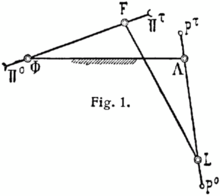An antiparallelogram linkage with one of its long edges ΦΛ fixed as a base. The two points F and L move in circles of equal radius but opposite direction.

For both the parallelogram and antiparallelogram linkages, if one of the long (crossed) edges of the linkage is fixed as a base, the free joints move on equal circles, but in a parallelogram they move in the same direction with equal velocities while in the antiparallelogram they move in opposite directions with unequal velocities. As James Watt discovered, if an antiparallelogram has its long side fixed in this way it forms a variant of Watt's linkage, and the midpoint of the unfixed long edge will trace out a lemniscate or figure eight curve. For the antiparallelogram formed by the sides and diagonals of a square, it is the lemniscate of Bernoulli.

The antiparallelogram is an important feature in the design of Hart's inversor, a linkage that (like the Peaucellier–Lipkin linkage) can convert rotary motion to straight-line motion. An antiparallelogram-shaped linkage can also be used to connect the two axles of a four-wheeled vehicle, decreasing the turning radius of the vehicle relative to a suspension that only allows one axle to turn. A pair of nested antiparallelograms was used in a linkage defined by Alfred Kempe as part of his universality theorem stating that any algebraic curve may be traced out by the joints of a suitably defined linkage. Kempe called the nested-antiparallelogram linkage a "multiplicator", as it could be used to multiply an angle by an integer.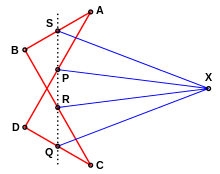Antiparallelogram braced to stop it turning into a normal parallelogram. The points PQRS are the midpoints of the sides and are collinear, and X can be any distance away on the perpendicular bisector of SQ. With this linkage AS2+SX2=AP2+PX2.

Without bracing an antiparallelogram linkage can be turned into a normal parallelogram. It can be braced to stop this happening using a construction by Abbott and Barton 2004. This construction can be used to fix a problem in Kempe's Universality Theorem.

## Celestial mechanics

In the n-body problem, the study of the motions of point masses under Newton's law of universal gravitation, an important role is played by central configurations, solutions to the n-body problem in which all of the bodies rotate around some central point as if they were rigidly connected to each other. For instance, for three bodies, there are five solutions of this type, given by the five Lagrangian points. For four bodies, with two pairs of the bodies having equal masses, numerical evidence indicates that there exists a continuous family of central configurations, related to each other by the motion of an antiparallelogram linkage.

## ReferencesWikimedia Commons has media related to Antiparallelograms.
1. Demaine, Erik; O'Rourke, Joseph (2007), Geometric Folding Algorithms, Cambridge University Press, pp. 32–33, ISBN 978-0-521-71522-5.
2. Bryant, John; Sangwin, Christopher J. (2008), "3.3 The Crossed Parallelogram", How round is your circle? Where Engineering and Mathematics Meet, Princeton University Press, pp. 54–56, ISBN 978-0-691-13118-4.